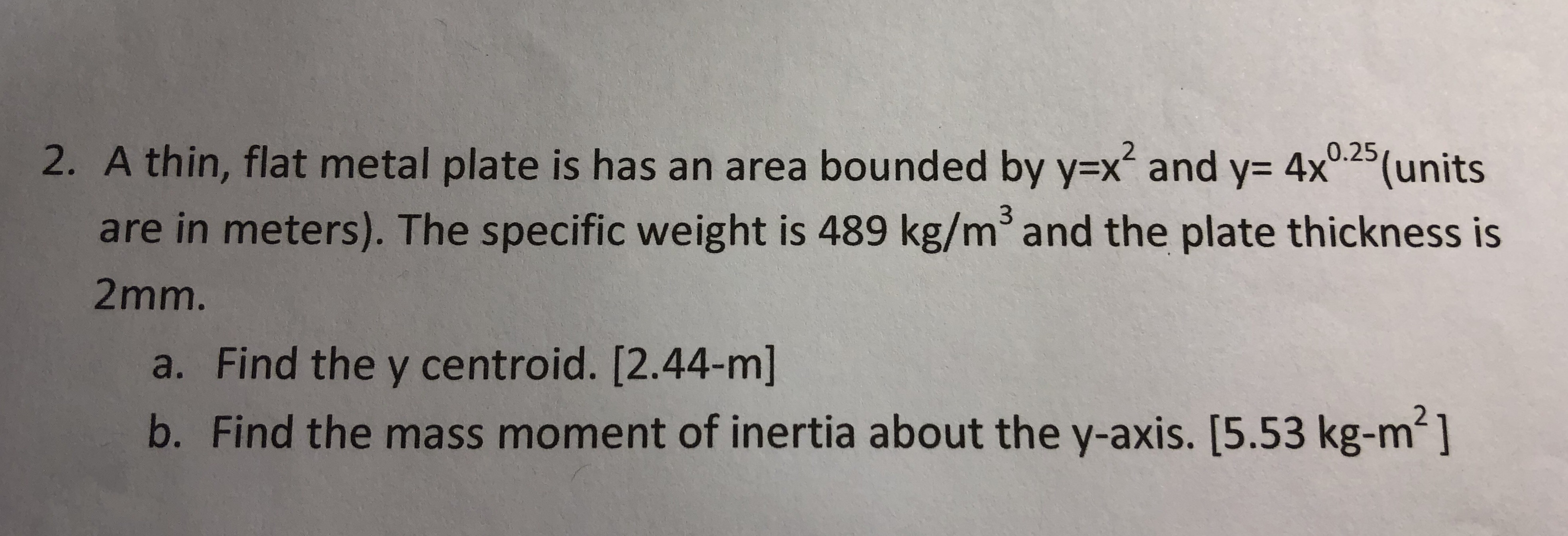2. A thin, flat metal plate is has an area bounded by yx and y- 4x025(unitsare in meters). The specific weight is 489 kg/m3and the plate thickness is2mm.a. Find the y centroid. [2.44-m]b. Find the mass moment of inertia about the y-axis. [5.53 kg-m2]

Questionhelp_outlineImage Transcriptionclose2. A thin, flat metal plate is has an area bounded by yx and y- 4x025(units are in meters). The specific weight is 489 kg/m3and the plate thickness is 2mm. a. Find the y centroid. [2.44-m] b. Find the mass moment of inertia about the y-axis. [5.53 kg-m2] fullscreen
Step 1

(a)   The given equation,

Step 2

Find the intersection of the given curves from (I) and (II),

Step 3

The expression for the area between the curves,

...

Want to see the full answer?

See Solution

Want to see this answer and more?

Our solutions are written by experts, many with advanced degrees, and available 24/7

See Solution
Tagged in

Mechanical Properties of Matter